Goddard Space Flight CenterPerhaps the most intriguing advance in X-ray astronomy instrumentation in the 1990s has been the development of the microcalorimeter, spearheaded by work at NASA's Goddard Space Flight Center.

What is a Microcalorimeter?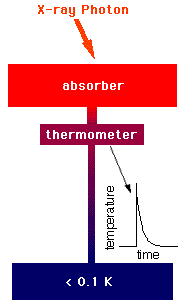A microcalorimeter is basically a thermal device made of an absorber, a thermistor, and a heat sink. The absorber must do 3 things: absorb X-rays from space efficiently, quickly, completely convert the absorbed energy into heat (thermalize the energy), and have a low heat capacity. There is no material known which excels at all 3 of these properties, so choosing the absorber material involves deciding on the best combination of them. The absorber in ASTRO-E's XRS instrument is HgTe (mercury-telluride) which is a compromise between good thermalization properties and low heat capacity. Other materials are better at absorbing X-rays while still having a low heat capacity, but those tested did not provide sufficient thermalization. A thermistor is a device that changes its electrical resistance dramatically with a small change in temperature. Since a thermometer is any device that measures temperature, a thermistor is a kind of thermometer.

The combination of the absorber and the thermistor is what we call the "X-ray detector". Below, starting from the left, is a diagram of a detector, a photo of a detector array (they are the gray-green rectangles in the middle), and a closeup of the array. You can (barely) see two black legs from each detector at the top of the image, and one leg from each detector squeezing between a pair of detectors at the bottom. These legs of the detector are what is called the "weak link" to the heat sink.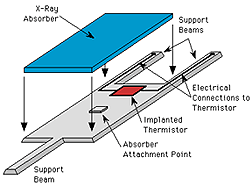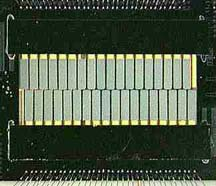The heat sink is what absorbs heat from the detector, keeping it cool. In the case of the XRS, the heat sink used to keep the detector cool enough to work is a refridgeration unit called the Adiabatic Demagnetization Refrigerator (ADR). Inside. the ADR is a special "pill" of salt that does the actual heat absorption.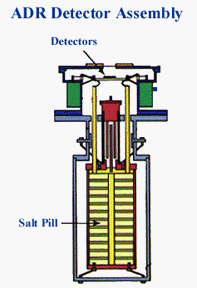How Does a Microcalorimeter Work?

 An X-ray photon hits the absorber and knocks an electron loose from an atom of the absorber material. This photoelectron (so-called because a photon of light knocked it loose) rattles around in the absorber, ultimately raising the temperature of the absorber by a few milliKelvin (that is, a few thousandths of one degree Kelvin). The temperature-sensitive thermistor is partially isolated from the absorber, to give the absorber time to come into equilibrium before the thermistor begins to see the temperature rise. After a few milliseconds, the thermistor comes to the same temperature as the absorber, a few milliKelvin warmer than the heat sink, which is at 65 milliKelvin. We know it's a little strange to be talking about 'heat' when something is near absolute zero! Next, the thermistor begins to cool as the heat flows out the weak link (the "legs" of the detector) to the heat sink. After a few tens of milliseconds, the thermistor has returned to its normal operating temperature.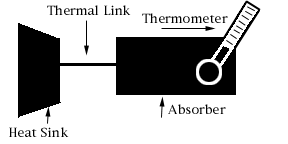The temperature rise (delta T) measured by the thermistor is approximately proportional to the energy of the X-ray photon:

delta T ~ E/C

where delta T is the change in temperature, E is the energy of the X-ray and C is the heat capacity of the absorber. So by measuring how much the temperature changes, we can determine the energy of the X-ray.

The Science

When an X-ray stops in a detector, it gives all of its energy to one electron. That electron can rattle around in the detector and give energy to other electrons. All these excited electrons would rather go back to their original energy. They want to return to what is called the 'ground state'. Through scattering with other electrons or with vibrations in the solid itself, they can lose that extra energy. But that energy has to go somewhere. What it does is heat the solid and increase its temperature. If you measure the change in temperature, you can measure how much energy the X-ray originally had.

How are heat and energy and temperature all related? Heat is a manifestation of energy. Heat and energy are measured in the same units (Joules). We usually think of energy as heat if we are thinking of the total energy of a large ensemble of objects that can exchange energy with each other and come into equilibrium. So, when an X-ray photon heats a solid, it gives its energy to the whole solid. On average, each atom is vibrating a little bit more than before the X-ray hit. Temperature is the way we relate the total energy of a system to its state of disorder (entropy). A physical property called "heat capacity" tells us how much the temperature rises in a material if we put in a certain amount of energy.

Suppose we wanted to measure the temperature increase due to an X-ray photon being absorbed. We'd want a very sensitive thermometer, something that had some physical property that changed a lot for a small change in temperature. And we'd want the detector to have a small heat capacity, so its temperature would change a lot for a small change in energy. And you'd want to do the whole thing at very low temperatures, because at room temperature there would already be too much thermal energy in your detector to see the very small change in energy from the X-ray. That is what an X-ray calorimeter does. It uses a silicon thermistor which has an electrical resistance which changes dramatically with small changes in temperature. It has a low heat capacity. And it operates at less than 0.1 K. That's Kelvin. Zero on the Kelvin scale is an absolute zero and represents the cessation of all thermal vibrations. Water freezes at 273 K. Nitrogen liquifies at 77 K. Helium liquifies at 4 K. and we operate calorimeters at less than 0.1 K! Calorimeters are able to get the best spectral resolution of any non-dispersive spectrometer.

 Using the text, and any external printed references, define the following terms: Kelvin, thermistor, heat capacity.

Reference URLs:

Thermodynamics
http://www.unidata.ucar.edu/staff/blynds/tmp.htmlA service of the High Energy Astrophysics Science Archive Research Center (HEASARC), Dr. Alan Smale (Director), within the Astrophysics Science Division (ASD) at NASA/GSFC